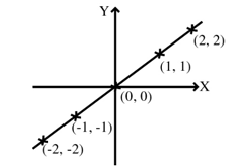# Identity Function

A function f from a set A to the same set A is said to be an identity function if f(x) = x for all x ∈ A i.e. f : A → A is defined by f(x) = x for all x ∈ A. Identity function is denoted by IA or I. Therefore, I(x) = x always.

Graph of Identity Function

The graph of the identity function f(x) = x is the graph of the function y = x. It is the straight line y = x.